Filter By

## All Questions

#### Two reactions R1 and R2 have identical pre-exponential factors.  Activation energy of R1 exceeds that of R2 by 10 kJ mol−1.  If k1 and k2 are rate constants for reactions R1 and R2 respectively at 300 K, then ln(k2/k1) is equal to : (R=8.314 J mol−1K−1)   Option: 1 6 Option: 2 4 Option: 3 8 Option: 4 12Taking log both the sides## Crack CUET with india's "Best Teachers"

• HD Video Lectures
• Unlimited Mock Tests
• Faculty Support#### Four resistances of  respectively in cyclic order to form Wheatstone's network. The resistance that is to be connected in parallel with the resistance of  to balance the network is ___________. Option: 1 10 Option: 2 5 Option: 3 15 Option: 4 20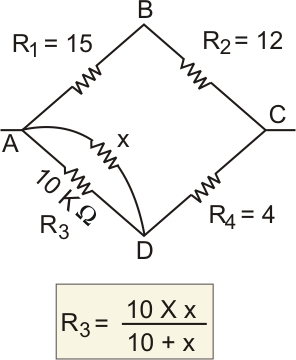For balanced Wheatstone bridge

As

So using

We get

let we connected x-ohms in parallel to 10-ohm resistance

i.e

we get

So the answer will be 10.

#### The length of a potentiometer wire is  and it carries a current of . For a cell of emf  and internal resistance of , the null point on it is found to be at . The resistance of whole wire is :   Option: 1 100 Option: 2 80 Option: 3 604 Option: 7 120

In the potentiometer, a battery of known emf  E is connected in the secondary circuit. A constant current I is flowing through AB from the driver circuit. The jockey is a slide on potentiometer wire AB to obtain null deflection in the galvanometer. Let   be the length at which galvanometer shows null deflection.

Since the potential of wire AB (V) is proportional to the length AB(L).

Similarly

So we get

given current through potentiometer wire =60mA

V=iR

So the correct option is 4.

## Crack NEET with "AI Coach"

• HD Video Lectures
• Unlimited Mock Tests
• Faculty Support#### A proton with kinetic energy of  moves from south to north. It gets acceleration of  by an applied magnetic field (west to east). The value of magnetic field ( in mT):  (Rest mass of a proton is ) Option: 1 0.71 Option: 2 71 Option: 3 0.071 Option: 4 7.1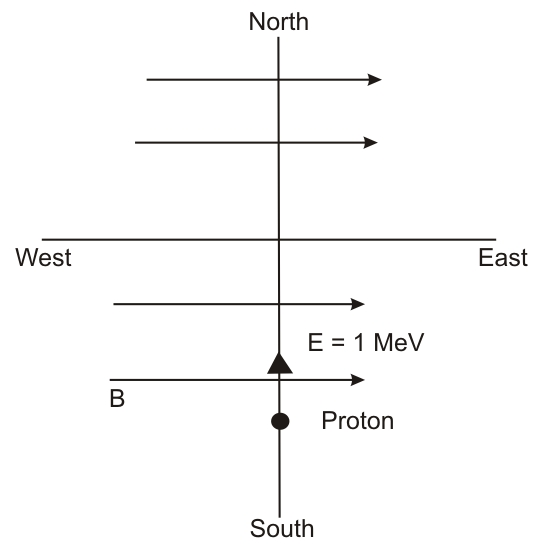So option (1) is correct

#### In a meter bridge experimental S is a standard resistance, R is resistance wire. It is found that balancing length is l=25 cm. If R is replaced by a wire of half length and half diameter that of R of same material, then the balancing distance(in cm) will now be _____.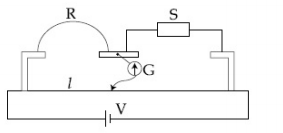Option: 1 40 Option: 2  50 Option: 3 20 Option: 4 30then,## Crack JEE Main with "AI Coach"

• HD Video Lectures
• Unlimited Mock Tests
• Faculty Support#### Two identical capacitors A and B, charged to the same potential 5V are connected in two different circuits as shown below at time t=0. If the charge on capacitors A and B at time t=CR isandrespectively , then (Here e is the base of natural logarithm)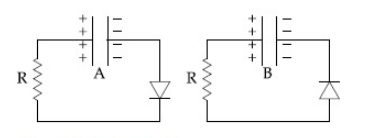Option: 1Option: 2Option: 3Option: 4Maximum charge on the capacitor(a) is reverse biased and (b) is forward biased

(a)                                                           (b)So,Hence the correct option is (4).

#### A small circular loop of conducting wire has radius a and carries current I. It is placed in a uniform magnetic field B perpendicular to its plane such that when it rotated slightly about its diameter and released, it starts performing simple harmonic motion of time period T. If the mass of the loop is m then : Option: 1Option: 2Option: 3Option: 4Hence the correct option is (4).

## Crack CUET with india's "Best Teachers"

• HD Video Lectures
• Unlimited Mock Tests
• Faculty Support#### The current I1 (in A) flowing through  resistor in the following circuit is:-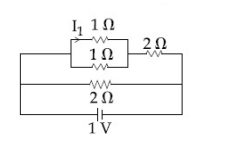Option: 1 0.25  Option: 2 0.40 Option: 3 0.2   Option: 4 0.5

Ohms Law -

1. Ohm’s law

In a conductor, if all external physical conditions like temperature and pressure are kept constant the Current flowing through a conductor is directly proportional to the Potential difference across two ends.

Electric Resistance

• The graph between V and IThe slope gives the resistance

• The graph between V and I at different temperaturesHere T1>T2. The resistance of a conductor increases with increase in temperature

-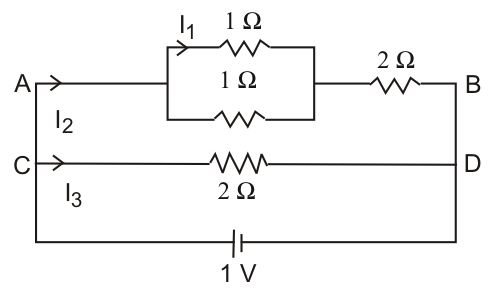As

and

From equation (1) and (2)

and

( when considered up to two decimal point)

Or,

(when considered up to one decimal point)

#### An electron gun is placed inside a long solenoid of radius R on its axis. The solenoid has n turns / length and carries a current I. The electron gun shoots an electron along the radius of the solenoid with speed. If the electron does not hit the surface of the solenoid, maximum possible value ofis (all symbols have their standard meaning) :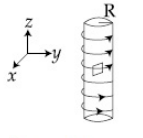Option: 1Option: 2Option: 3Option: 4Malus's Law -

Malus's Law-

This law states that the intensity of the polarized light transmitted through the analyzer varies as the square of the cosine of the angle between the plane of transmission of the analyzer and the plane of the polarizer.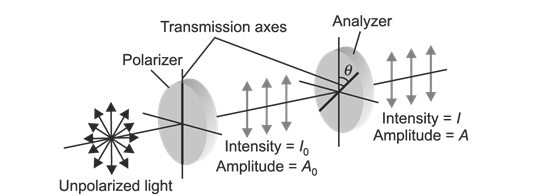As,

output intensity is given by

Initial output intensity=10% of I_0

I.e

Final output intensity=O

means new angle is

the angle by which the analyser needs to be rotated further is

So Option (3) is correct.